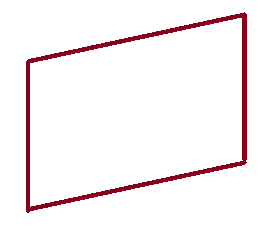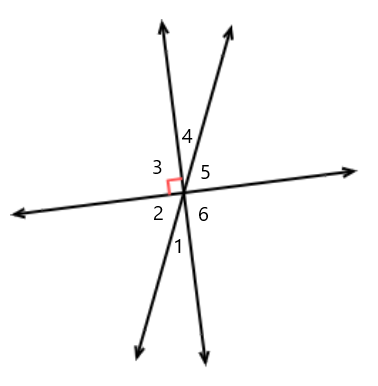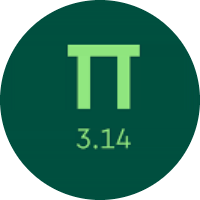# Efficient Learning of Two Dimensional Figures

In this quiz, you will learn about two-dimensional figures. You will learn about regular and irregular polygons, parallel, perpendicular, intersecting lines, types of angles, and the properties of polygons.

Start Quiz

What name best describes this shape?rectangle

square

parallelogram

Is a rhombus always a quadrilateral?

yes

no

One angle of an isosceles triangle measures 90°. Which other angle can be in that isosceles triangle?

45°

90°

35°

65°

A right-angle triangle has one angle that measures 65°. What is the measure of another acute angle?

15°

35°

25°

20°

One angle of a triangle measures 45°. The other two angles are in a ratio of 8:19. What are the measures of those two angles?

30° and 105°

35° and 100°

45° and 90°

40° and 95°

One angle of a parallelogram measures 55°. What are the measures of the other three angles in the parallelogram?

55°,125°,125°

65°,115°,115°

45°,135°,135°

What is ∠m?116°

88°

98°

What is the sum of the measures of the angles in a pentagon?

540°

450°

360°

Which angle is complementary to ∠1?∠4

∠3

∠6

∠5

The measure of an angle is 25°. What is the measure of its supplementary angle?

135°

145°

155°

180°

Quiz/Test Summary
Title: Efficient Learning of Two Dimensional Figures
Questions: 10
Contributed by: